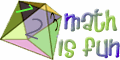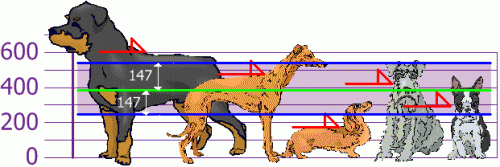# Autarchy of the Private Cave

## R functions for regression analysis cheat sheet

29th May 2012

## Information criteria for choosing best predictive models

29th May 2012

Usually I’m using 10-fold (non-stratified) CV to measure the predictive power of the models: it gives consistent results, and is easy to perform (at least on smaller datasets).

Just came across the Akaikeâ€™s InforÂ­maÂ­tion Criterion (AIC) and Schwarz Bayesian InforÂ­maÂ­tion Criterion (BIC). Citing robjhyndman,

AsympÂ­totÂ­iÂ­cally, minÂ­iÂ­mizÂ­ing the AIC is equivÂ­aÂ­lent to minÂ­iÂ­mizÂ­ing the CV value. This is true for any model (Stone 1977), not just linÂ­ear modÂ­els. It is this propÂ­erty that makes the AIC so useÂ­ful in model selecÂ­tion when the purÂ­pose is prediction.

Because of the heavÂ­ier penalty, the model choÂ­sen by BIC is either the same as that choÂ­sen by AIC, or one with fewer terms. AsympÂ­totÂ­iÂ­cally, for linÂ­ear modÂ­els minÂ­iÂ­mizÂ­ing BIC is equivÂ­aÂ­lent to leaveâ€“vâ€“out cross-â€‹â€‹validation when v = n[1-1/(log(n)-1)] (Shao 1997).

Want to try AIC and maybe BIC on my models. Conveniently, both functions exist in R.

Posted in Bioinformatics, Machine learning | No Comments »

29th March 2010

Posted in Bioinformatics, Links, Science, Systems Biology | 1 Comment »

## Standard deviation and variance in pictures

24th January 2010MathIsFun offers nicely illustrated pages on math, algebra, geometry and maybe more.

For example, there is a step-by-step instruction on calculating variance and standard deviation for a set of measured dog heights, with a final picture (below) illustrating one-sigma distance from the mean. Unfortunately, concepts of normal distribution and %% of data points within each sigma range are not discussed, but that might as well be too much for a nice explanation. There are also animations, like this mean machine. Overall, MathIsFun is a nice resource for younglings.## Mean, standard deviation, and stem-and-leaf plot

14th September 2006

I am doing some simple statistics now, and had to review some basic concepts like standard deviation.
As a note to myself and anyone interested, here it stays.

The mean is just a sum of all your numerical observations, divided by the number of observations. E.g., if you have measured how tall your 5 children are, and got the values 1.42, 1.56, 1.05, 1.89, 1.92, the “mean height” of your children will be x = (1.42 + 1.56 + 1.05 + 1.89 + 1.92) / 5, x = 7.84 / 5 = 1.568 (all values in metres).

The mean itself doesn’t tell you much, however. If you had this 1.568 mean available, you wouldn’t know even the range of heights.

The standard deviation helps with this. First of all, it is measured in the same units as initial data – i.e. metres in our example. Second, it gives you an idea of how strongly do the measured values differ in your sample – the bigger the deviation, the longer is the value range measured in the sample.
Read the rest of this entry »

Posted in Science | No Comments »